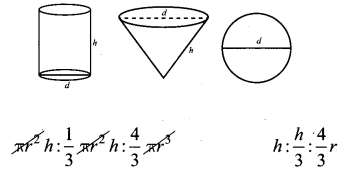## Tamilnadu Samacheer Kalvi 10th Maths Solutions Chapter 7 Mensuration Ex 7.5

Multiple choice questions.
Question 1.
The curved surface area of a right circular cone of height 15 cm and base diameter 16 cm is ______
(1) 60π cm2
(2) 68π cm2
(3) 120π cm2
(4) 136π cm2
(4) 136π cm2
Hint: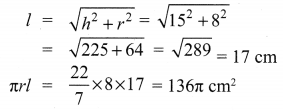Question 2.
If two solid hemispheres of same base radius r units are joined together along with their bases, then the curved surface area of this new solid is
(1) 4πr2 sq. units
(2) 67πr2 sq. units
(3) 3πr2 sq. units
(4) 8πr2 sq. units
Solution:
(1) 47πr2 sq. units]Question 3.
The height of a right circular cone whose radius is 5 cm and slant height is 13 cm will be __________
(1) 12 cm
(2) 10 cm
(3) 13 cm
(4) 5 cm
(1) 12 cm
Hint: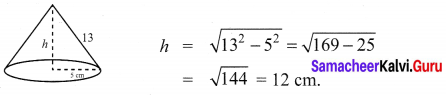Question 4.
If the radius of the base of a right circular cylinder is halved keeping the same height, then the ratio of the volume of the cylinder thus obtained to the volume of original cylinder is
(1) 1 : 2
(2) 1 : 4
(3) 1 : 6
(4) 1 : 8
Solution:
(2) 1 : 4Question 5.
The total surface area of a cylinder whose radius is $$\frac{1}{3}$$ of its height is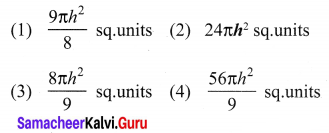Solution:
(3) $$\frac{8 \pi h^{2}}{9}$$ sq. units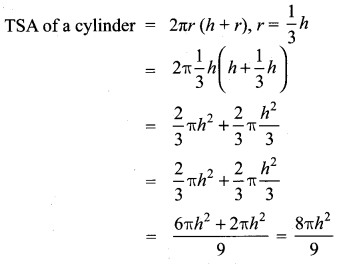Question 6.
In a hollow cylinder, the sum of the external and internal radii is 14 cm and the width is 4 cm. If its height is 20 cm, the volume of the material in it is _______
(1) 560π cm3
(2) 1120π cm3
(3) 56π cm3
(4) 360π cm3
(2) 1120π cm3
Hint:
R + r = 14 cm
w = 4 cm
h = 90 cm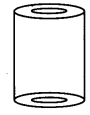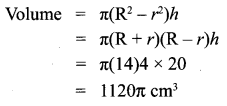Question 7.
If the radius of the base of a cone is tripled and the height is doubled then the volume is
(4) unchanged
Solution:
Hint: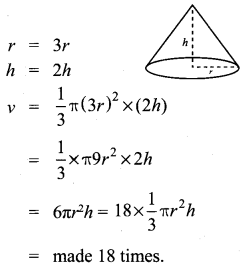Question 8.
The total surface area of a hemisphere is how many times the square of its radius ______
(1) π
(2) 4π
(3) 3π
(4) 2π
(3) 3π
Hint: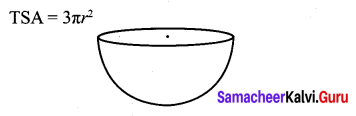Question 9.
A solid sphere of radius x cm is melted and cast into a shape of a solid cone of same radius. The height of the cone is
(1) 3x cm
(2) x cm
(3) 4x cm
(4) 2x cm
Solution:
(3) 4x cm
Hint: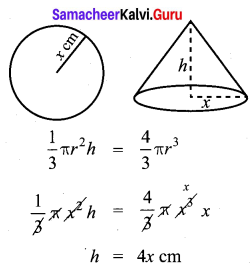Question 10.
A frustum of a right circular cone is of height 16 cm with radii of its ends as 8 cm and 20 cm. Then, the volume of the frustum is _______
(1) 3328π cm3
(2) 3228π cm3
(3) 3240π cm3
(4) 3340π cm3
(1) 3328π cm3Hint: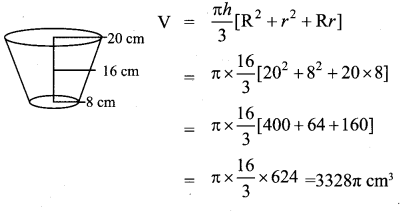Question 11.
A shuttlecock used for playing badminton has the shape of the combination of
(1) a cylinder and a sphere
(2) a hemisphere and a cone
(3) a sphere and a cone
(4) frustum of a cone and a hemisphere
Solution:
(4) frustum of a cone and a hemisphere

Question 12.
A spherical ball of radius r1 units is melted to make 8 new identical balls each of radius r2 units. Then r1 : r2 is
(1) 2 : 1
(2) 1 : 2
(3) 4 : 1
(4) 1 : 4
Solution:
Hint: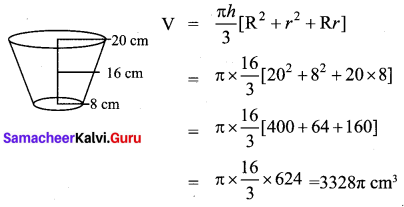Question 13.
The volume (in cm3) of the greatest sphere that can be cut off from a cylindrical log of wood of base radius 1 cm and height 5 cm is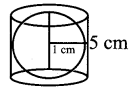Solution:
(1) $$\frac{4}{3} \pi$$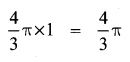Question 14.
The height and radius of the cone of which the frustum is a part are h1 units and r1 units respectively. Height of the frustum is h2 units and the radius of the smaller base is r2 units. If h2: h1 = 1 : 2 then r2 : r1 is
(1) 1 : 3
(2) 1 : 2
(3) 2 : 1
(4) 3 : 1
Solution:
(2) 1 : 2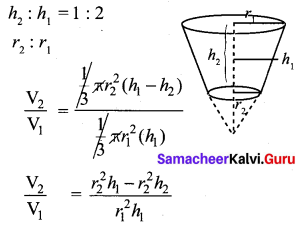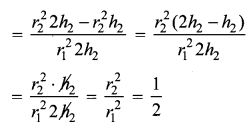Question 15.
The ratio of the volumes of a cylinder, a cone and a sphere, if each has the same diameter and same height is ____
(1) 1 : 2 : 3
(2) 2 : 1 : 3
(3) 1 : 3 : 2
(4) 3 : 1 : 2